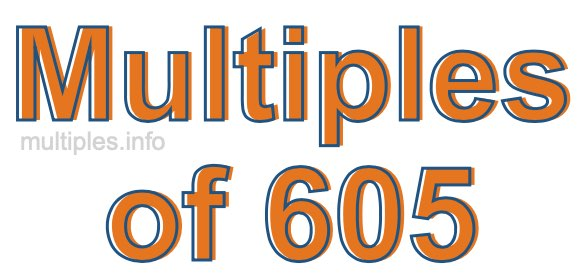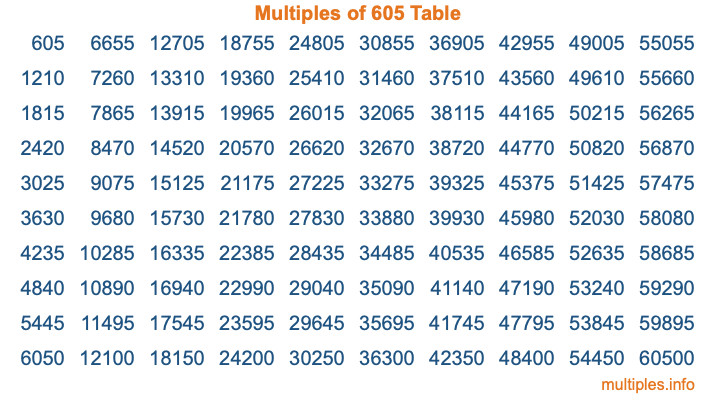Multiples of 605Welcome to the Multiples of 605 page. Here we will first teach you everything you will ever need to know about the multiples of 605, and then give you a study guide summary of everything we taught you to make sure you remember it all. Use this page to look up facts and learn information about the multiples of 605. This page will make you a multiples of six hundred five expert!

Definition of Multiples of 605
Multiples of 605 are all the numbers that when divided by 605 equal an integer. Each of the multiples of 605 are called a multiple. A multiple of 605 is created by multiplying 605 by an integer.

Therefore, to create a list of multiples of 605, you start with 1 multiplied by 605, then 2 multiplied by 605, then 3 multiplied by 605, and so on for as long as you want. Thus, the list of the first five multiples of 605 is 605, 1210, 1815, 2420, and 3025. To see a larger list of multiples of 605, see the printable image of Multiples of 605 further down on this page. We also have a category where you can choose any nth multiple of 605.

Multiples of 605 Checker
The Multiples of 605 Checker below checks to see if any number of your choice is a multiple of 605. In other words, it checks to see if there is any number (integer) that when multiplied by 605 will equal your number. To do that, we divide your number by 605. If the the quotient is an integer, then your number is a multiple of 605.

Is  a multiple of 605?

Least Common Multiple of 605 and ...
A Least Common Multiple (LCM) is the lowest multiple that two or more numbers have in common. This is also called the smallest common multiple or lowest common multiple and is useful to know when you are adding our subtracting fractions. Enter one or more numbers below (605 is already entered) to find the LCM.

Check out our LCM Calculator if you need more details about the Least Common Multiple or if you need the LCM for different numbers for adding and subtraction fractions.

nth Multiple of 605
As we stated above, 605 is the first multiple of 605, 1210 is the second multiple of 605, 1815 is the third multiple of 605, and so on. Enter a number below to find the nth multiple of 605.

th multiple of 605

Multiples of 605 vs Factors of 605
605 is a multiple of 605 and a factor of 605, but that is where the similarities end. All postive multiples of 605 are 605 or greater than 605. All positive factors of 605 are 605 or less than 605.

Below is the beginning list of multiples of 605 and the factors of 605 so you can compare:

Multiples of 605: 605, 1210, 1815, 2420, 3025, etc.

Factors of 605: 1, 5, 11, 55, 121, 605

As you can see, the multiples of 605 are all the numbers that you can divide by 605 to get a whole number. The factors of 605, on the other hand, are all the whole numbers that you can multiply by another whole number to get 605.

It's also interesting to note that if a number (x) is a factor of 605, then 605 will also be a multiple of that number (x).

Multiples of 605 vs Divisors of 605
The divisors of 605 are all the integers that 605 can be divided by evenly. Below is a list of the divisors of 605.

Divisors of 605: 1, 5, 11, 55, 121, 605

The interesting thing to note here is that if you take any multiple of 605 and divide it by a divisor of 605, you will see that the quotient is an integer.

Multiples of 605 Table
Below is an image of the first 100 multiples of 605 in a table. The table is in chronological order, column by column. The first column has the first ten multiples of 605, the second column has the next ten multiples of 605, and so on.The Multiples of 605 Table is also referred to as the 605 Times Table or Times Table of 605. You are welcome to print out our table for your studies.

Negative Multiples of 605
Although not often discussed or needed in math, it is worth mentioning that you can make a list of negative multiples of 605 by multiplying 605 by -1, then by -2, then by -3, and so on, to get the following list of negative multiples of 605:

-605, -1210, -1815, -2420, -3025, etc.

Multiples of 605 Summary
Below is a summary of important Multiples of 605 facts that we have discussed on this page. To retain the knowledge on this page, we recommend that you read through the summary and explain to yourself or a study partner why they hold true.

There are an infinite number of multiples of 605.

A multiple of 605 divided by 605 will equal a whole number.

605 divided by a factor of 605 equals a divisor of 605.

The nth multiple of 605 is n times 605.

The largest factor of 605 is equal to the first positive multiple of 605.

605 is a multiple of every factor of 605.

605 is a multiple of 605.

A multiple of 605 divided by a divisor of 605 equals an integer.

605 divided by a divisor of 605 equals a factor of 605.

Any integer times 605 will equal a multiple of 605.

Multiples of a Number
Here you can get the multiples of another number, all with the same attention to detail as we did for multiples of 605 on this page.

Multiples of
Multiples of 606
Did you find our page about multiples of six hundred five educational? Do you want more knowledge? Check out the multiples of the next number on our list!